Next: Two-Dimensional Incompressible Inviscid Flow Up: Incompressible Inviscid Flow Previous: Irrotational Flow

# Exercises

1. Liquid is led steadily through a pipeline that passes over a hill of height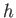into the valley below, the speed at the crest being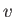. Show that, by properly adjusting the ratio of the cross-sectional areas of the pipe at the crest and in the valley, the pressure may be equalized at these two places. (Milne-Thomson 1958.)

2. Water of mass density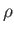and pressure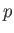flows through a curved pipe of uniform cross-sectional area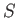, whose radius of curvature is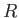, at the uniform speed. Demonstrate that there is a net force per unit lengthacting on the pipe, and that this force is everywhere directed away from the pipe's local center of curvature. Here,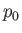is atmospheric pressure.

3. Water is held in a right circular conical tank whose apex lies vertically below the center of its base. The water initially fills the tank to a heightabove the vertex. Letbe the initial radius of the surface of the water inside the tank. A small hole of area(that is much less than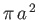) is made at the bottom of the tank. Demonstrate that the time required to empty the tank is at least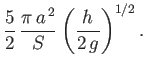4. Water is held in a spherical tank of radius, and initially fills the tank to a height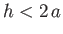above its lowest point. A small hole of area(that is much less than) is made at the bottom of the tank. Demonstrate that the time required to empty the tank is at least5. Water is held in two contiguous tanks whose cross-sectional areas,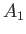and, are independent of height. A small hole of area(where,) is made in the wall connecting the tanks. Assuming that the initial difference in water level between the two tanks is, show that the time required for the water levels to equilibrate is at least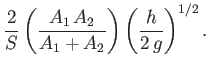6. For a channel of width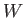, having a discharge rate, show that there is a critical depth, where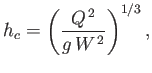which must be exceeded before a hydraulic jump is possible.

7. Show that for a stationary hydraulic jump in a rectangular channel, the upstream Froude number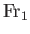, and the downstream Froude number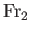, are related by8. Consider a simply-connected volumewhose boundary is the surface. Suppose thatcontains an incompressible fluid whose motion is irrotational. Let the velocity potentialbe constant over. Prove thathas the same constant value throughout. [Hint: Consider the identity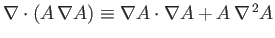.]

9. In Exercise 8, suppose that, instead oftaking a constant value on the boundary, the normal velocity is everywhere zero on the boundary. Show thatis constant throughout.

10. An incompressible fluid flows in a simply-connected volumebounded by a surface. The normal flow at the boundary is prescribed. Show that the flow pattern with the lowest kinetic energy is irrotational. This result is known as the Kelvin minimum energy theorem. [Hint: Try writing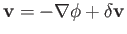, whereis the velocity potential of the irrotational flow pattern. Letthroughout, andon. Show that the kinetic energy is lowest when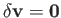throughout.]Next: Two-Dimensional Incompressible Inviscid Flow Up: Incompressible Inviscid Flow Previous: Irrotational Flow
Richard Fitzpatrick 2016-03-31